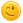# You will be given 3 integers as input The inputs may or may not be different from each other.

You will be given 3 integers as input. The inputs may or may not be
different from each other.

You have to output 1 if all three inputs
are different from each other,
and 0 if any input is repeated more
than once.

answered Jul 31, 2019 by (280 points)
#include<stdio.h>

int main()

{

int a,b,c;

printf("enter the numbers:  ");

scanf("%d%d%d",a,b,c);

if(a!=b&&b!=c&&c!=a)

{

printf("1");

}

else{

printf("0");

}

}
answered Jul 31, 2019 by (300 points)
#include<stdio.h>

int main()

{

int a,b,c,k=0,r=0;

scanf("%d%d%d",&a,&b,&c);

if(a!=b&&b!=c&&c!=a)

k=1;

else

r++;

if(k==1&&r==0)

printf("0");

else

printf("1");

return 0;

}
answered Jul 31, 2019 by (140 points)

#include<stdio.h>
int main()
{
int a,b,c;
scanf("%d%d%d",&a,&b,&c);
if(a==b&&a==c)
printf("0");
else
printf("1");
}

commented Aug 1, 2019 by anonymous
This fails if b and c are equal but a isn't.
answered Jul 31, 2019 by (160 points)
#include <stdio.h>

int main ()

{
int a, b, c;
printf ("Enter three numbers to be compared: ");  //asking for input
scanf ("%d%d%d", &a, &b, &c);  //Taking input

if (a == b || b == c)   //Comparing as per condition
{
printf ("0");  //output case 1
}
else
{
printf("1");  //output case 2
}

}
commented Aug 1, 2019 by anonymous
This fails if a and c are equal
answered Aug 1, 2019 by (1,600 points)

#include <stdio.h>
void main()
{
int a=0, b=0, c=0;
printf("%c",(a==b)?'0':(c==a)?'0':(c==b)?'0':'1',scanf("%d%d%d",&a,&b,&c));
}

Try this one.

answered Aug 1, 2019 by anonymous
bool func(int a, int b, int c){

return a^b^c;

}
commented Aug 9, 2019 by (1,600 points)
_Bool f(int a, int b, int c){
return a^b&&a^c&&b^c;
}
this would be the correct answer i guess.
answered Aug 5, 2019 by (220 points)
#include<stdio.h>
void main()
{
int a,b,c;
scanf("%d %d %d",&a,&b,&c);
if(a==b||a==c||b==c)
printf("1");
else
printf("0");
}